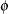Default Estimation Techniques

Based on the structure of the model, the GLIMMIX procedure selects the estimation technique for estimating the model parameters. If you fit a generalized linear mixed model, you can change the estimation technique with the METHOD= option in the PROC GLIMMIX statement. The defaults are determined as follows:

• generalized linear model

• normal distribution: restricted maximum likelihood

• all other distributions: maximum likelihood

• generalized linear model with overdispersion
Parameters (;, if present) are estimated by (restricted) maximum likelihood as for generalized linear models. The overdispersion parameter is estimated from the Pearson statistic after all other parameters have been estimated.

• generalized linear mixed models
The default technique is METHOD=RSPL, corresponding to maximizing the residual log pseudo-likelihood with an expansion about the current solutions of the best linear unbiased predictors of the random effects. In models for normal data with identity link, METHOD=RSPL and METHOD=RMPL are equivalent to restricted maximum likelihood estimation, and METHOD=MSPL and METHOD=MMPL are equivalent to maximum likelihood estimation. This is reflected in the labeling of statistics in the "Fit Statistics" table.